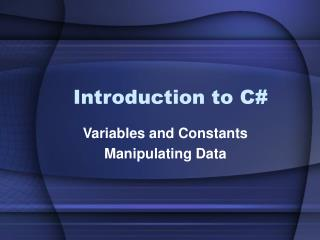DownloadDownload PresentationIntroduction to C#

# Introduction to C#

Download Presentation## Introduction to C#

- - - - - - - - - - - - - - - - - - - - - - - - - - - E N D - - - - - - - - - - - - - - - - - - - - - - - - - - -
##### Presentation Transcript

1. Introduction to C# Variables and Constants Manipulating Data

2. Agenda • Different Types of Data • Choosing the Right Type • Constants • Manipulating Data • Conditional Expressions

3. The Need for Data • Computer programs manipulate data • There is a need to store data • Variables: • Represent a named cell in the computer’s memory • Used to hold information • When you declare a variable: • You open up some of the computer’s memory • You’re giving the variable an identifier (name) • You only do this once per variable

4. Different Data Types • A data type describes the kind of information a variable can hold • Some data types are built into the language (intrinsic) • Some data types we define ourselves(user-defined) • Different Forms: • Primitive data types • Complex data types (made from primitive data types)

5. Intrinsic Data Types • Byte (8 bits) • Whole numbers: • short(-32768 to 32767) • int (-2,147,483,648 to 2,147,483,647) • long(±9,223,372,036,854,775,808) • In .NET, these don’t change size regardless of language or OS!

6. Signed and Unsigned • Each whole-number type can be signed (positive and negatives) or unsigned (only positives) • Preface the type with “u” • ushort (0 to 65535) • uint (0 to 4,294,967,295) • ulong (0 to 0xFFFFFFFFFFFFFFFF)

7. Intrinsic Data Types (cont) • Decimal numbers • float (±1.5*1045 to ±3.4*1038 with 7 significant digits) • double (±5.0*10324 to ±1.8*10308 with 15-16 significant digits) • decimal (fixed-precision with 28 significant digits) – used for financials

8. Other Data Types • char holds a character • Denoted by single quotes • Escape sequences denoted with a backslash (i.e. ‘\n’ for newline) • string • Denoted by double quotes • string myString = “Something clever”;

9. The Right Type • Which type do you pick? • Depends on the data you want to store • Most of the time, an int or float will do for numbers • Why have all these other types? • (hint: what other things can we program for?)

10. Declaring Variables • Format: <data type> <variable name>; • Variable name you can choose almost anything • Examples: byte age; float gpa; string name; char letterGrade; • Now that they’re declared • Don’t need to re-declare them • Can refer to them by their name

11. Legal Variable Names • Can’t be a reserved word • Can’t begin with a number • Can’t contain special symbols • Like #, @, !, ^, &, /, ), or SPACES • Exceptions: underscore _, and dollar sign \$ • Examples: byte \$theValue; // legal char test_value; // legal double double; // not legal int rum&coke; // not legal bool true or false; // not legal for two reasons!

12. Assignment • Variables are used to store values • Use the = operator • All variables must be initialized (given a starting value) • Example: int myInt = 0; int yourInt = myInt; char myChar = ‘c’; float myFloat = 6.5f;

13. Operators • + adds two variables/literals together (including Strings) • - subtraction • / division • * multiplication • % modulus (remainder) • ( and ) follows standard math rules • Example: int result = ( (4 % 3) * 5) + 1; // result gets 6 • Note: the code result + 1 by itself does NOT change the value of result (no = operator)

14. Converting Types • Called ‘type casting’ • Hitting it ‘with a big hammer’ • When you are trying to put something ‘larger’ into something ‘smaller’ • Example char c = (char)65; // Assign an ‘A’ int myInt = 6; short s = (short)myInt; // put an int into a short! float myFloat = (float)6.5;

15. Shortcuts • ++ will add one to the current value of a variable int counter = 5; int counter = 5; counter++; counter = counter +1; • += will modify the variable by a certain amount int counter = 5; int counter = 5; counter += 3; counter =counter + 3; Same as Same as

16. Constants • A constant is a variable that doesn’t change • Define using the const operator • Example: const double PI = 3.1415926;

17. Conclusion • Variables are easy to declare • There are several intrinsic types • Initialize your variables! • You may have to type cast during assignment